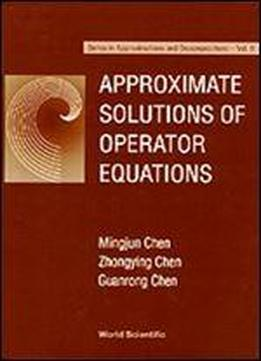# Approximate Solutions Of Operator Equations by Mingjun Chen / 1997 / English / PDF

This book offers an elementary and self-contained introduction to many fundamental issues concerning approximate solutions of operator equations formulated in an abstract Banach space setting, including important topics such as solvability, computational schemes, convergence, stability and error estimates. The operator equations under investigation include various linear and nonlinear types of ordinary and partial differential equations, integral equations, and abstract evolution equations, which are frequently involved in applied mathematics and engineering applications.Each chapter contains well-selected examples and exercises, for the purposes of demonstrating the fundamental theories and methods developed in the text and familiarizing the reader with functional analysis techniques useful for numerical solutions of various operator equations.

views: 495